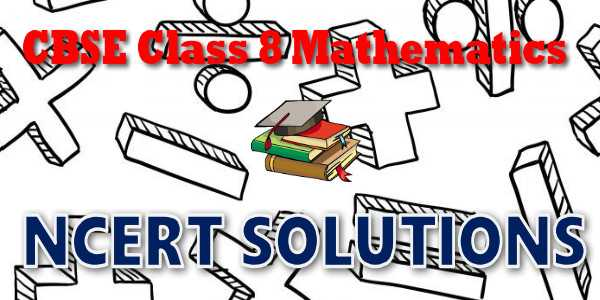No products in the cart.

# CBSE - Class 08 - Mathematics - Understanding Quadrilaterals - NCERT Solutions## CBSE, JEE, NEET, NDA

Question Bank, Mock Tests, Exam Papers

NCERT Solutions, Sample Papers, Notes, Videos

## NCERT Solutions for Mathematics Understanding Quadrilaterals## myCBSEguide App

Complete Guide for CBSE Students

NCERT Solutions, NCERT Exemplars, Revison Notes, Free Videos, CBSE Papers, MCQ Tests & more.

# NCERT solutions for class 8 Mathematics## NCERT Class 8 Mathematics Chapter-wise Solutions

Maths Part I

• Chapter 1 - Rational Numbers
• Chapter 2 - Linear Equations in One Variable
• Chapter 3 - Understanding Quadrilaterals
• Chapter 4 - Practical Geometry
• Chapter 5 - Data Handling
• Chapter 6 - Squares and Square Roots
• Chapter 7 - Cubes and Cube Roots
• Chapter 8 - Comparing Quantities
• Chapter 9 - Algebraic Expressions and Identities
• Chapter 10 - Visualising Solid Shapes
• Chapter 11 - Mensuration
• Chapter 12 - Exponents and Powers
• Chapter 13 - Direct and Inverse Proportions
• Chapter 14 - Factorisation
• Chapter 15 - Introduction to Graphs
• Chapter 16 - Playing with Numbers

## CBSE class 8th Mathematics have two books. Each book has chapters and topics.

• NCERT Mathematics Book Class 8 Part-1

Here is the list of topics covered under each chapter of class 8 Mathematics NCERT text book.

• Exercise 1.1
• Exercise 1.2

• Exercise 2.1
• Exercise 2.2
• Exercise 2.3
• Exercise 2.4
• Exercise 2.5
• Exercise 2.6

• Exercise 3.1
• Exercise 3.2
• Exercise 3.3
• Exercise 3.4

• Exercise 4.1
• Exercise 4.2
• Exercise 4.3
• Exercise 4.4
• Exercise 4.5

• Exercise 5.1
• Exercise 5.2
• Exercise 5.3

• Exercise 6.1
• Exercise 6.2
• Exercise 6.3
• Exercise 6.4

• Exercise 7.1
• Exercise 7.2

• Exercise 8.1
• Exercise 8.2
• Exercise 8.3

• Exercise 9.1
• Exercise 9.2
• Exercise 9.3
• Exercise 9.4
• Exercise 9.5

### 10. Visualising Solid Shapes

• Exercise 10.1
• Exercise 10.2
• Exercise 10.3

### 11. Mensuration

• Exercise 11.1
• Exercise 11.2
• Exercise 11.3
• Exercise 11.4

### 12. Exponents and Powers

• Exercise 12.1
• Exercise 12.2

### 13. Direct and Inverse Proportions

• Exercise 13.1
• Exercise 13.2

### 14. Factorisation

• Exercise 14.1
• Exercise 14.2
• Exercise 14.3
• Exercise 14.4

### 15. Introduction to Graphs

• Exercise 15.1
• Exercise 15.2
• Exercise 15.3

### 16.Playing with Numbers

• Exercise 16.1
• Exercise 16.2

## NCERT Solutions Chapter 3 Understanding Quadrilaterals

You know that the paper is a model for a plane surface. When you join a number of points without lifting a pencil from the paper (and without retracing any portion of the drawing other than single points), you get a plane curve

Try to recall different varieties of curves you have seen in the earlier classes. Match the following: (Caution!Afigure may match to more than one type).

(a) Simple closed curve

(b) A closed curve that is not simple

(c) Simple curve that is not closed

(c) Simple curve that is not closed

## NCERT Solutions for Class 8th Mathematics

NCERT Solutions Class 8 Mathematics PDF (Download) Free from myCBSEguide app and myCBSEguide website. Ncert solution class 8 Mathematics includes text book solutions from  part . NCERT Solutions for CBSE Class 8 Mathematics have total 16 chapters. Class 8 Mathematics ncert Solutions in pdf for free Download are given in this website. Ncert Mathematics class 8 solutions PDF and Mathematics ncert class 8 PDF solutions with latest modifications and as per the latest CBSE syllabus are only available in myCBSEguide.

## NCERT Solutions for Class 5

NCERT Solutions for Class 4

## NCERT Solutions for Class 3## myCBSEguide

Trusted by 1 Crore+ Students

#### Question Paper Creator

• Create papers in minutes
• Print with your name & Logo